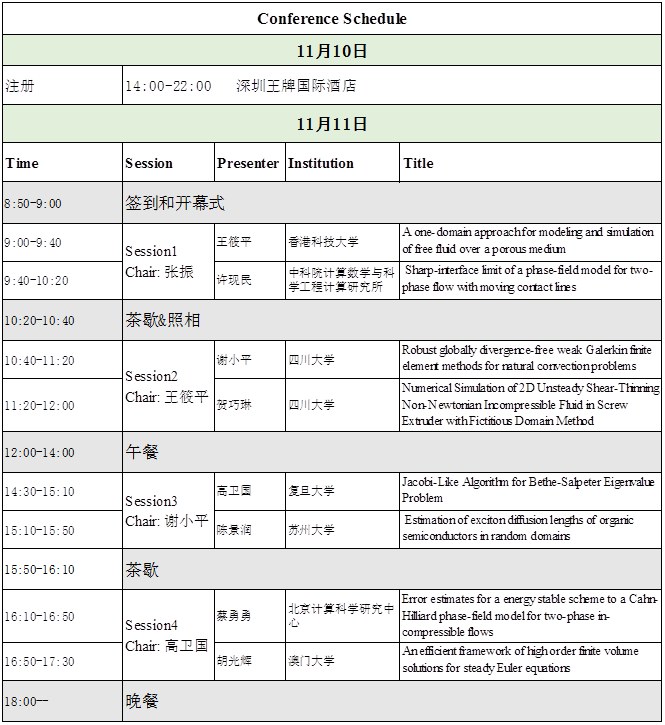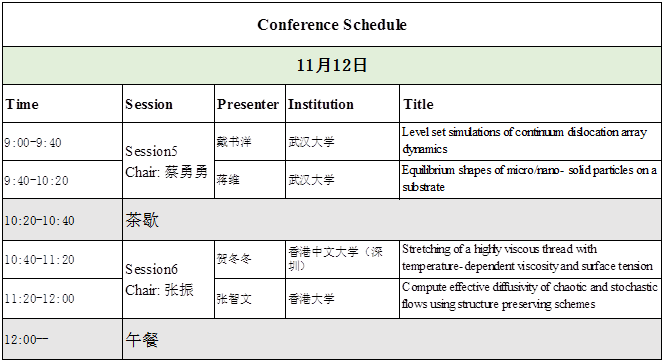# 流体力学和材料科学中的计算问题

2017/11/10-2017/11/12

1.会议议题

近年来计算数学方法在流体力学以及材料科学问题的研究中展现越来越重要的地位，促进该领域研究飞速的进展。为了加强这一交叉学科领域研究各个理论方法和模型应用的同行之间的进一步学术交流，促进相关领域研究的发展，由南方科技大学数学系主办的“流体力学和材料科学中的计算问题”将于20171110日至12日在南方科技大学（深圳市南山区西丽学苑大道1088号）召开。会议的主题包括流体力学与材料科学中的数学模型和数值计算方法的最新研究成果和研究动态，内容涉及包括多相流、流固耦合、位错、固态去湿、有机半导体等问题的多尺度建模和高精度数值算法。

2.学术委员会

3.参会人员

4.会议时间安排

5.住宿安排及交通指引

1)深圳北站

A.  至深圳王牌国际酒店

B．至南方科技大学

2) 宝安国际机场

A．至深圳王牌国际酒店

B．至南方科技大学

滴滴或出租：约45分钟80

6.联系人

7.会议日程Abstracts

Speaker: Jingrun Chen
Title: Estimation of exciton diffusion lengths of organic semiconductors in random domains
Abstract: Exciton diffusion length plays a vital role in the function of opto-electronic devices. Oftentimes, the domain occupied by a organic semiconductor is subject to surface measurement error. In many experiments, photoluminescence over the domain is measured and used as the observation data to estimate this length parameter in an inverse manner based on the least square method. However, the result is sometimes found to be sensitive to the surface geometry of the domain. We propose an asymptotic-based method  as an approximate forward solver whose accuracy is justified both theoretically and numerically. It only requires to solve several deterministic problems over a fixed domain. Therefore, for the same accuracy requirement we tested here, the running time of our approach is more than one order of magnitude smaller than that of directly solving the original stochastic boundary-value problem by the stochastic collocation method. In addition, from numerical results we find that the correlation length of randomness is important to determine whether a 1D reduced model is a good surrogate. This is joint work with Ling Lin (Sun Yat-Sen University), Zhiwen Zhang (University of Hong Kong), and Xiang Zhou (City University of Hong Kong).

Speaker: Yongyong Cai
Title: Error estimates for a energy stable scheme to a Cahn-Hilliard phase-field model for two-phase in-compressible flows
Abstract: We carry out r a rigorous error analysis for  a finite element  discretization of the   linear, weakly coupled  energy stable  scheme  for a Cahn-Hilliard phase-field model of two-phase incompressible flows with matching density.

Speaker: Shuyang Dai
Title: Level set simulations of continuum dislocation array dynamics
Abstract: We present a simulation method for the dynamics of dislocation arrays. In this numerical method, dislocation arrays are considered as continuous surfaces in three dimensions, and the level set representation is used for these dislocation array surfaces. The driving force of the evolution of the dislocation array surfaces comes from both the long-range interaction of the constituent dislocations and their local curvature effect. The long-range interaction, which is expressed by a complicated integral over the whole dislocation array surface, is calculated efficiently using the fast Fourier transform (FFT) method. Simulations are performed for dislocation arrays bypassing different particles under applied stress and are compared with those of a single dislocation. The long-range nature of the stress fields of nonplanar infinite dislocation arrays is discussed, and is shown to be essentially different from that by a single dislocation.

Speaker: Weiguo Gao
Title: Jacobi-Like Algorithm for Bethe-Salpeter Eigenvalue Problem
Abstract: In this talk, we investigate the Bethe-Salpeter eigenvalue problems and propose a Jacobi-like algorithm. The eigen-structure is preserved during the iterations. Global convergence of the new algorithm is given and numerical examples demonstrate the fast convergence. This is a joint-work with Meiyue Shao and Chenming Yang.

Speaker: Dongdong He
Title: Stretching of a highly viscous thread with temperature-dependent viscosity and surface tension
Abstract: In this talk, I will show our recent results for the extension of the highly viscous threads arising from the glass and polymer industrial processing.  We consider the evolution of a long and thin vertically-aligned axisymmetric viscous thread, which is attached to a solid wall at its upper end, experiences gravity and is pulled at its lower end by a fixed force. The thread experiences either heating or cooling by its environment. Both the viscosity and surface tension are assumed to be functions of temperature. A set of one-dimensional model is derived through the formal slender body asymptotic analysis. When inertia is completely neglected and the temperature of the environment is spatially uniform, we obtain analytic solutions for an arbitrary initial shape and temperature profile. In addition, we determine the criteria for whether the cross-section of a given fluid element will ever become zero and hence determine the minimum stretching force that is required for pinching. For non-zero Reynolds numbers, we show that the dynamics is subtly influenced by inertia and the pinching location is selected by a competition between three distinct mechanisms. In particular, for a thread with initially uniform radius and a spatially uniform environment but with a non-uniform initial temperature profile, pinching can occur either at the hottest point, at the points near large thermal gradients or at the pulled end, depending on the Reynolds number.

Speaker: Qiaolin He
Title: Numerical Simulation of 2D Unsteady Shear-Thinning Non-Newtonian Incompressible Fluid in Screw Extruder with Fictitious Domain Method
Abstract: We develop a fictitious domain method with Distributed Lagrange Multipliers for simulating 2D unsteady shear-thinning non-newtonian incompressible flow in a single-screw and twin-screw extruder. The advantage of the fictitious domain method is that one can use a fixed mesh even as the fluid domain changes in time (as the screws are rotating), eliminating the need for repeated remeshing and projection. The method uses a finite element discretization in space and an operator-splitting technique for discretization in time. Numerical results are given for the flow inside a single-screw extruder or twin-screw extruder, which show the fictitious domain method is efficient for the problem.

Speaker: Guanghui Hu
Title: An efficient framework of high order finite volume solutions for steady Euler equations
Abstract: An efficient solver for steady Euler equations is an essential component in optimal design problems, which is a class of important applications in the compressible computational fluid dynamics. In this talk, we will discuss the necessity on developing high order methods, describe our high order finite volume framework for steady Euler equations, and introduce the methods we used for improving the efficiency. A variety of numerical experiments show the effectiveness of our method.

Speaker: Wei Jiang
Title: Equilibrium shapes of micro/nano-solid particles on a substrate.
Abstract: We present a new approach for predicting stable equilibrium shapes of crystalline islands on flat substrates, as commonly occur through solid-state dewetting of thin films. The new theory is a generalization of the widely used Winterbottom construction, i.e., an extension of the Wulff construction for particles on substrates). This approach is equally applicable to cases where the crystal surface energy is isotropic, weakly anisotropic, strongly anisotropic and ``cusped''. We demonstrate that, unlike in the classical Winterbottom approach, multiple equilibrium island shapes may be possible when the surface energy is strongly anisotropic. We analyze these shapes through perturbation analysis, by calculating the first and second variations of the total free energy functional with respect to contact locations and island shape. Based on this analysis, we find the necessary conditions for the equilibria to be stable to two-dimensional perturbations and exploit this through a generalization of the Winterbottom construction to identify all possible stable equilibrium shapes. Finally, we propose a dynamical evolution method based on surface diffusion mass transport to determine whether all of the stable equilibrium shapes are dynamically accessible. Applying this approach, we demonstrate that islands with different initial shapes may evolve into different stationary shapes and show that these dynamically-determined stationary states correspond to the predicted stable equilibrium shapes, as obtained from the generalized Winterbottom construction.

Speaker: Xiao-Ping Wang
Title: A one-domain approach for modeling and simulation of free fluid over a porous medium
Abstract: We propose a one-domain approach based on the Brinkman model for the modeling and simulation of the transport phenomenon between free fluid and a porous medium. A thin transition layer is introduced between the free fluid region and the porous media region, across which the porosity and permeability undergo a rapid but continuous change. We study the behavior of the solution to the one-domain model analytically and numerically. Using the method of matched asymptotic expansion, we recover the Beavers–Joseph–Saffman (BJS) interface condition as the thickness of the transition layer goes to zero. We also calculate the error estimates between the leading order solution of the one-domain model and the standard Darcy–Stokes model of two-domain model with BJS condition. Numerical methods are developed for both the one-domain model and the two-domain model. Numerical results are presented to support the analytical results, thereby justifying the one-domain model as a good approximation to the two domain Stokes–Darcy model.

Speaker: Xiaoping Xie
Title: Robust globally divergence-free weak Galerkin finite element methods for natural convection problems
Abstract: We develop a class of Weak Galerkin finite element methods for natural convection problems.  We use the \$P_k/P_{k-1}/P_k\$ \$(k\geq 1)\$ discontinuous finite element combination for the approximation of velocity, pressure and temperature, and piecewise \$P_l/P_k/P_l (l = k-1, k)\$ for the numerical traces of velocity, pressure and temperature. Our methods yield globally divergence-free velocity solutions. Error estimates are derived, and numerical results are provided to demonstrate the robustness of the methods.  This is a joint work with Yihui Han.

Speaker: Xianmin Xu
Title: Sharp-interface limit of a phase-field model for two-phase flow with moving contact lines
Abstract: Moving contact line is a classical problem that falls beyond the framework of conventional hydrodynamics. Standard no-slip boundary condition leads to infinite energy dissipation. To overcome the difficulties, many models are proposed. One of them is proposed by T. Qian, X.-P. Wang & S. Sheng, which is a phase-field model that can describe slipping of the contact line on solid boundary due to uncompensated Young stress and is consistent the molecular dynamics simulations. In this talk, we will show some recent study on the sharp-interface limit of the model. By asymptotic analysis, we show that the model will converge to different sharp-interface limits under different choices for the mobility parameter and a relaxation parameter in the boundary condition. Numerical results are consistent with the analysis results and also illustrate the different convergence rates of the sharp-interface limits for different scalings of the two parameters. This is a joint work with Yana Di and Haijun Yu.

Speaker: Zhiwen Zhang
Title: Compute effective diffusivity of chaotic and stochastic flows using structure preserving schemes
Abstract: In this talk we study the problem of computing the effective diffusivity for a particle moving in chaotic and stochastic flows. In addition we numerically investigate the residual diffusion phenomenon in chaotic advection. Instead of solving the Fokker-Planck equation in the Eulerian formulation, we compute the motion of particles in the Lagrangian formulation, which is modelled by stochastic differential equations (SDEs). We propose a new numerical integrator based on a stochastic splitting method to solve the corresponding SDEs. We provide some error analysis for the new numerical integrator using the backward error analysis technique and show that our method outperforms standard Euler-based integrators. Numerical results are presented to demonstrate the accuracy and efficiency of the proposed method for several typical chaotic and stochastic flow problems of physical interests.